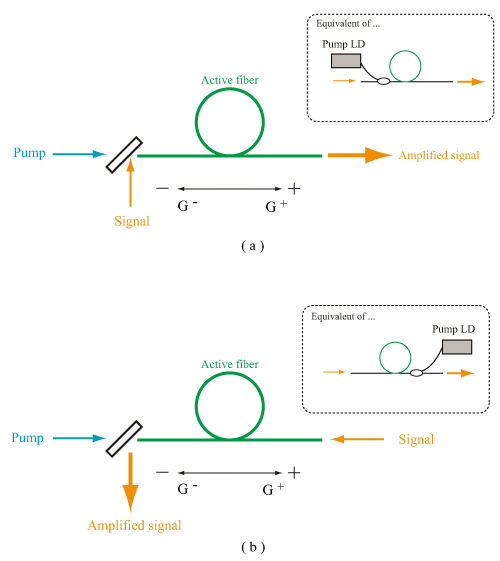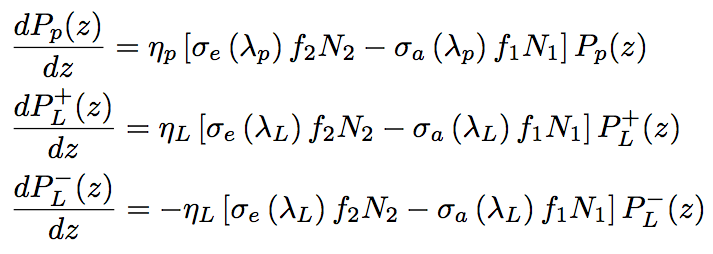By deriving an equation of light propagation in an optical fiber for forward- and backward-pumped system, shown in Fig. (a) and (b), respectively, here we estimate a small-signal gain of optical fiber amplifier.Fig. Schematic of (a) forward-pumped and (b) backward-pumped systems

When PL+, signal light propagating in the forward direction for pumping light in the forward-pumped system, and PL, signal light propagating in the opposite direction for pumping light in the backward pumping system are given, propagation equations for signal and pumping light (Pp) along the optical axis of fiber are expressed as follows,where f1 is a Yb3+ ratio in L1 state to the ground state, and f2 is a Yb3 ratio in U0 state to the excited state. The first, and second term in the first equation corresponds to a process that Yb3+ transfers to the lower state of laser medium by stimulated emission, and a process that Yb3+ is excited to the upper state. In the second and third equations, the first, and second terms correspond to the stimulated emission processes of signal light, and the absorption processes of signal light. ηp, and ηL means an overlapping rate of pumping, and signal light with fiber core, respectively. For a double-clad fiber, ηp, ηL is approximately Aco/Acl, 1, respectively, where Aco, and Acl is a cross-section area of fiber core and internal clad, respectively. These equations enable numerical analysis of amplification gain and laser output power.